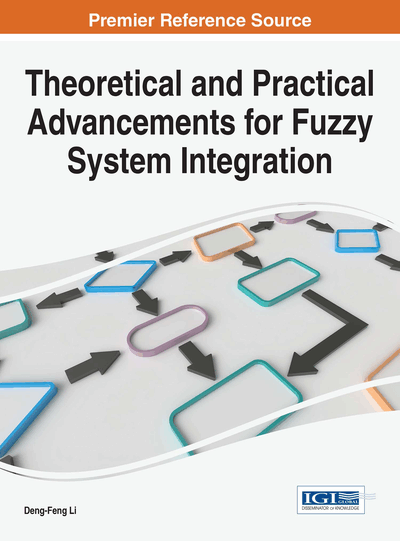# Credibility Hypothesis Testing of Variance of Fuzzy Normal Distribution

S. Sampath (University of Madras, India) and B. Ramya (University of Madras, India)
Copyright: © 2017 |Pages: 28
DOI: 10.4018/978-1-5225-1848-8.ch008
OnDemand PDF Download:
Available
\$37.50
No Current Special Offers

## Abstract

This paper considers the problem of developing test procedures for testing credibility hypotheses about the variance of fuzzy normal distribution assuming the expected values of the distributions mentioned under null and alternative credibility hypotheses are known and equal. The cases where the underlying hypothesis is simple and composite (one sided) are considered. Tests have been derived with the help of the membership ratio criterion. Properties possessed by the developed tests, like best credibility rejection region and uniformly best rejection region have been studied. Examples are also given to illustrate the usage of the derived tests.
Chapter Preview
Top

## Introduction

A first study on Credibility Theory which is an analogue of Probability Theory to study subjective uncertainty has been carried out by Liu and Liu (2002). Credibility theory is considered as an important branch of mathematics. Further refinements on Credibility theory has been carried out by Liu (2008). Researchers have considered two different dimensions of Credibility theory, namely,

• Theoretical and

• Applied aspects.

Theoretical developments such as:

• Inequalities of fuzzy variables,

• Concept of convergence,

• Fundamental theorems,

• Independence of fuzzy variables,

• Fuzzy dominance and

• The concept of entropy, have been studied by various researchers.

On the other hand, the theory related to credibility theory has been applied in practical situations. For example, a study of:

• Modeling parallel machine schedule problem,

• Vehicle routing problem,

• Project scheduling problem,

• Quantity inventory model,

• Vendor selection problem in a supply chain were considered by a group of researchers.

Sampath and Ramya (2015) have a given a brief listing of works done in connection with applications of Credibility Theory. Sampath and Vaidyanathan (2009), Sampath and Kalaivani (2010) and Sampath and Senthilkumar (2014) applied credibility theory in mining of fuzzy datasets. Sampath (2009) and Sampath and Deepa (2013) considered the application of credibility theory in fuzzy statistical quality control.1Wan and Li (2013) and Wan et al. (2013) used Triangular intuitionistic fuzzy numbers to deal with ill-known quantities in decision data and decision making problems.

Huge volume of works has been done on theoretical aspects of credibility theory. Even though several studies have been made, one can feel the dearth of studies on inferential aspects of credibility theory. However, some studies have been made in Uncertainty theory which is a theory closely related to credibility theory. Estimation of the parameters involved in Uncertainty distributions have been considered by Wang and Peng (2012), and Wang et al. (2012). Wang et al. (2012a) proposed the concept of testing of hypothesis under uncertain situations. Further, Sampath and Ramya (2013) introduced a distance based approach for testing Uncertainty hypothesis. Sampath and Ramya (2015) developed membership ratio criterion for the problem of testing hypothesis about credibility distributions and studied important properties of test yielded by the membership ratio criterion with reference to the parameters of triangular credibility distribution. Sampath and Ramya (2014) considered tests for testing hypothesis about the expected value of fuzzy normal distributions and identified their optimal properties assuming the variances under null and alternative hypotheses are known and equal. In this chapter, the question of developing credibility tests for testing hypothesis about the variance of fuzzy normal distribution is considered when the expected values of the distributions under null and alternative credibility hypotheses are equal and known. The cases where the null and alternative credibility hypotheses are simple as well as composite (one sided) are considered.

## Complete Chapter List

Search this Book:
Reset